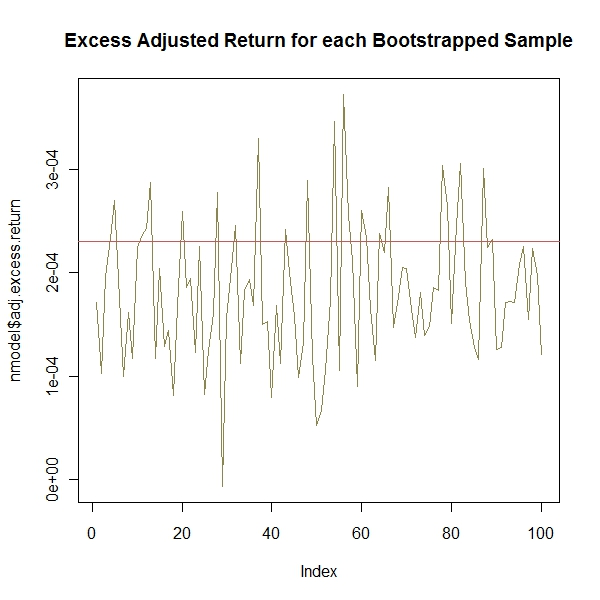Want to share your content on R-bloggers? click here if you have a blog, or here if you don't.

Just to remind everyone, THIS IS NOT INVESTMENT ADVICE AND ANY ACTIONS TAKEN BASED ON THIS DISCUSSION WILL PROBABLY RESULT IN SIGNIFICANT LOSSES.

We had fun with the ttrTests package in two previous posts ttrTests: Its Great Thesis and Incredible Potential and ttrTests Experimentation.  Let’s actually run some real tests on the basic CUD indicator introduced in A Quantstrat to Build On Part 6.  We will start by using paramStats to test multiple parameters.From TimelyPortfolio

After we find the best parameter, we will test with returnStats the actual returns using the best parameter for the CUD indicator versus a buy-and-hold approach.From TimelyPortfolio

Finally, we will try to apply some statistical rigidity to the process by using 100 bootstrapped samples to test the best parameter on out-of-sample data. As always, please let me know your thoughts.From TimelyPortfolioFrom TimelyPortfolioFrom TimelyPortfolio

`#let's define our silly countupdown function#as a sample of a custom ttr ruleCUD <- function(x,params=50,...) {	#CUD takes the n-period sum of 1 (up days) and -1 (down days)	temp <- ifelse(runSum(ifelse(ROC(x,1,type="discrete") > 0,1,-1),params)>=0,1,0)	#replace NA with 0 at beginning of period	temp[is.na(temp)] <- 0	temp}   require(ttrTests)require(quantmod)require(lattice)require(reshape2)require(PerformanceAnalytics)   #defaults functions is overridden by ggplot2 and plyr if loaded#and will cause problems if you want to use ttrTests concurrently   tckrs <- c("GSPC","RUT","N225","GDAXI","DJUBS")   #use 1 or GSPC but adjust however you would likei=1getSymbols(paste("^",tckrs[i],sep=""),from="1896-01-01",to=Sys.Date())test_price <- as.vector(get(tckrs[i])[,4])#do parameter tests but plot=FALSE#we will plot laterparam_results <- paramStats(x=test_price, ttr = CUD, start = 20, nSteps = 30, stepSize = 10,	restrict = FALSE, burn = 0, short = FALSE, condition = NULL,	silent = TRUE, TC = 0.001, loud = TRUE, plot = FALSE, alpha = 0.025,	begin = 1, percent = 1, file = "", benchmark = "hold")#make output slightly more usable with some naming#believe I got this rightnames(param_results) <- c("excess.return","z.score","adj.excess.return",	"Sharpe.ratio","best","best.repeat","best.adjusted",	paste("tested.parameters",c(1:(NROW(param_results)-7)),sep=""))#jpeg(filename="excess by parameter.jpg",	quality=100,width=6.25, height = 6.25,  units="in",res=96)plot(param_results\$excess.return~param_results\$tested.parameters1,	type="l", col="darkgray",	main="ttrTests Excess Return by Parameter")abline(v=param_results\$best, col="indianred3")#dev.off()   #let's use the returnStats function to get#more complete return and distribution info on the best parameterstats <- returnStats(x=test_price, ttr=CUD, params=param_results\$best,	short=FALSE, TC=0.001, benchmark="hold")#make output slightly more usable with some naming#believe I got this rightnames(stats) <- c("benchmark.stats","ttr.stats","adj.stats.and.periods",	"excess.stats","long.stats","short.stats","neutral.stats")#jpeg(filename="analysis of returns.jpg",	quality=100,width=6.25, height = 6.25,  units="in",res=96)barplot(c(stats\$long.stats,stats\$short.stats,	stats\$neutral.stats,stats\$benchmark.stats),	col=c("darkolivegreen3","indianred3","steelblue3","gray70"),	names.arg=c("long","short","neutral","benchmark"),	main="Analysis of Returns from ttrTests")#dev.off()   #now let's test the best parameter with nullModel#this tests the parameter with bootstrap resampling#for significance across one of three criteria#specified by crit = "sharpe", "return" (excess return),#or "adjust" (excess adjusted  for trading costs)nmodel <- nullModel(x=test_price, model="stationaryBootstrap", userParams=4, bSamples=100,	ttr=CUD, params=param_results\$best, short=FALSE, TC=0.001, crit="sharpe",	benchmark="hold")#make output slightly more usable with some naming#believe I got this right#this is different from documentation but code seems to#fit with thisnames(nmodel) <- c("excess.return","excess.Sharpe.ratio","adj.excess.return","p.value")#jpeg(filename="excess return by bootstrap.jpg",	quality=100,width=6.25, height = 6.25,  units="in",res=96)plot(nmodel\$excess.return,type="l",xlab="Bootstrap Sample",	main="Excess Return for each Bootstrapped Sample")#add line for excess return from actual ttr performanceabline(h=stats\$excess.stats, col="indianred3")#dev.off()   #jpeg(filename="excess adjusted return by bootstrap.jpg",	quality=100,width=6.25, height = 6.25,  units="in",res=96)plot(nmodel\$adj.excess.return,type="l",col="khaki4",	main="Excess Adjusted Return for each Bootstrapped Sample")#add line for excess adjusted from actual ttr performanceabline(h=stats\$adj.stats.and.periods, col="indianred3")#dev.off()   #jpeg(filename="excess sharpe ratio by bootstrap.jpg",	quality=100,width=6.25, height = 6.25,  units="in",res=96)plot(nmodel\$excess.Sharpe.ratio,type="l",lwd=2,xlab="BootstrapSample",col="cadetblue4",	main="Excess Sharpe Ratio for each Bootstrapped Sample")#add line for excess sharpe from actual ttr performanceabline(h=stats\$ttr.stats-stats\$benchmark.stats, col="indianred3")#dev.off()`